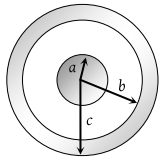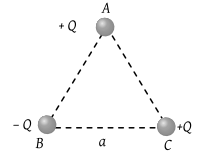The force between two charges 0.06 m apart is 5 N. If each charge is moved towards the other by 0.01 m, then the force between them will become

(1) 7.20 N

(2) 11.25 N

(3) 22.50 N

(4) 45.00 N

Concept Questions :-

Coulomb's law
High Yielding Test Series + Question Bank - NEET 2020

Difficulty Level:

When 1019 electrons are removed from a neutral metal plate, the electric charge on it is

(1) –1.6 C

(2) +1.6 C

(3) 10+19 C

(4) 10–19 C

Concept Questions :-

Ways of charging
High Yielding Test Series + Question Bank - NEET 2020

Difficulty Level:

Two charges each equal to 2μC are 0.5m apart. If both of them exist inside vacuum, then the force between them is

(1) 1.89 N

(2) 2.44 N

(3) 0.144 N

(4) 3.144 N

Concept Questions :-

Coulomb's law
High Yielding Test Series + Question Bank - NEET 2020

Difficulty Level:

A solid conducting sphere of radius a has a net positive charge 2Q. A conducting spherical shell of inner radius b and outer radius c is concentric with the solid sphere and has a net charge –Q. The surface charge density on the inner and outer surfaces of the spherical shell will be(1) $-\frac{2Q}{4\pi {b}^{2}},\frac{Q}{4\pi {c}^{2}}$

(2) $-\frac{Q}{4\pi {b}^{2}},\frac{Q}{4\pi {c}^{2}}$

(3) $0,\frac{Q}{4\pi {c}^{2}}$

(4) None of the above

Concept Questions :-

Gauss law
High Yielding Test Series + Question Bank - NEET 2020

Difficulty Level:

Three charges are placed at the vertices of an equilateral triangle of side ‘a’ as shown in the following figure. The force experienced by the charge placed at the vertex A in a direction normal to BC is(1) ${Q}^{2}/\left(4\pi {\epsilon }_{0}{a}^{2}\right)$

(2) $-{Q}^{2}/\left(4\pi {\epsilon }_{0}{a}^{2}\right)$

(3) Zero

(4) ${Q}^{2}/\left(2\pi {\epsilon }_{0}{a}^{2}\right)$

Concept Questions :-

Coulomb's law
High Yielding Test Series + Question Bank - NEET 2020

Difficulty Level:

Two particles of equal mass m and charge q are placed at a distance of 16 cm. They do not experience any force. The value of $\frac{q}{m}$ is

(1) l

(2) $\sqrt{\frac{\pi {\epsilon }_{0}}{G}}$

(3) $\sqrt{\frac{G}{4\pi {\epsilon }_{0}}}$

(4) $\sqrt{4\pi {\epsilon }_{0}G}$

Concept Questions :-

Coulomb's law
High Yielding Test Series + Question Bank - NEET 2020

Difficulty Level:

An electron is moving around the nucleus of a hydrogen atom in a circular orbit of radius r. The Coulomb force $\stackrel{\to }{F}$ on electron is (Where $K=\frac{1}{4\pi {\epsilon }_{0}}$)

(1) $-K\frac{{e}^{2}}{{r}^{3}}\stackrel{^}{r}$

(2) $K\frac{{e}^{2}}{{r}^{3}}\stackrel{\to }{r}$

(3) $-K\frac{{e}^{2}}{{r}^{3}}\stackrel{\to }{r}$

(4) $K\frac{{e}^{2}}{{r}^{2}}\stackrel{^}{r}$

Concept Questions :-

Coulomb's law
High Yielding Test Series + Question Bank - NEET 2020

Difficulty Level:

Five balls numbered 1 to 5 are suspended using separate threads. Pairs (1, 2), (2, 4), and (4, 1) show electrostatic attraction, while pair (2, 3) and (4, 5) show repulsion. Therefore ball 1 must be

(1) Positively charged

(2) Negatively charged

(3) Neutral

Concept Questions :-

Coulomb's law
High Yielding Test Series + Question Bank - NEET 2020

Difficulty Level:

Equal charges q are placed at the four corners A, B, C, D of a square of length a. The magnitude of the force on the charge at B will be

(1) $\frac{3{q}^{2}}{4\pi {\epsilon }_{0}{a}^{2}}$

(2) $\frac{4{q}^{2}}{4\pi {\epsilon }_{0}{a}^{2}}$

(3) $\left(\frac{1+2\sqrt{2}}{2}\right)\frac{{q}^{2}}{4\pi {\epsilon }_{0}{a}^{2}}$

(4) $\left(2+\frac{1}{\sqrt{2}}\right)\frac{{q}^{2}}{4\pi {\epsilon }_{0}{a}^{2}}$

Concept Questions :-

Coulomb's law
High Yielding Test Series + Question Bank - NEET 2020

Difficulty Level:

Two identical conductors of copper and aluminium are placed in an identical electric field. The magnitude of induced charge in the aluminum will be

(1) Zero

(2) Greater than in copper

(3) Equal to that in copper

(4) Less than in copper

Concept Questions :-

Electric field